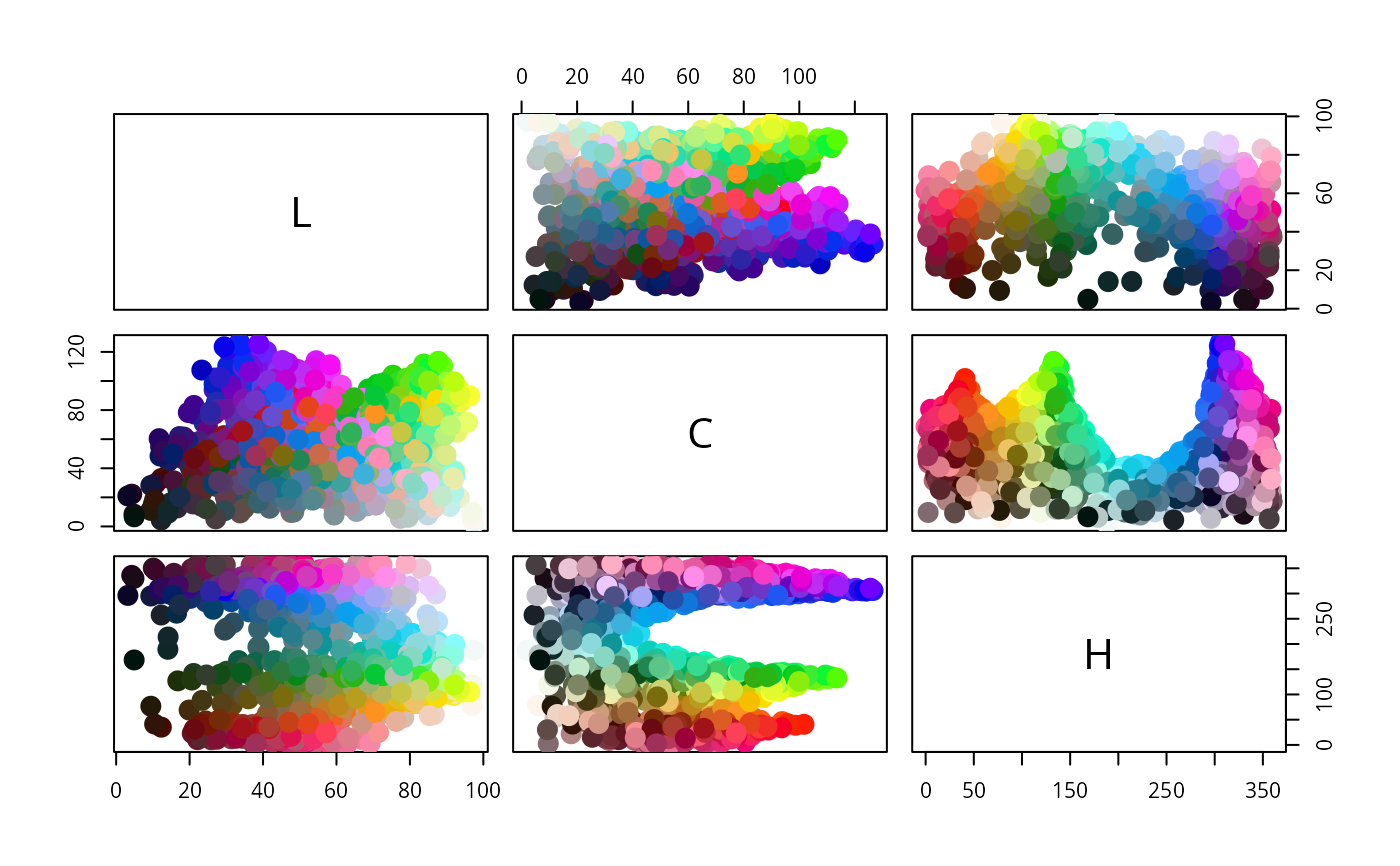This function creates colors of class polarLAB''; a subclass of the virtual color-class class.

polarLAB(L, C, H, names)

## Arguments

L, C, H these arguments give the L, C and H coordinates of the colors. The values can be provided in separate L, C and H vectors or in a three-column matrix passed as L. A vector of names for the colors (by default the row names of L are used).

## Value

An object of class polarLAB which inherits from class color.

## Details

The polarLAB space is a transformation of the CIE $$L^*a^*b^*$$ space so that the $$a$$ and $$b$$ values are converted to polar coordinates. The radial component $$C$$ measures chroma and the angular coordinate $$H$$ is measures hue.

RGB, HSV, XYZ, LAB, polarLAB, LUV, polarLUV.
## Show the polarLAB space
#> [1,] 76.00763 29.26965 260.1883plot(y)